# shell scripting: using if condition

Pranaam to all bhai ji _/\_
in this tutorial we are going to discuss about conditional statement 'if'
if is a conditional statement and commands/statements defined in the if condition will execute when condition defined for if statement is true .
before proceeding to this, we should go through some of mathematical expression symbols that we will use in tutorial

-eq   equal to
-ne   not equal to
-lt     less the
-le    less then equal to
-gt   greater then
-ge  greater then equal to

if
syntax for if .. then is
if  condition
then
statement 1
statement 2
statement3
....
....
statement n
fi

here condition is the condition which is the parameter for execution of statements written in if condition block
like, if you are feeling hungry, you will eat food
here hunger is the condition for eating food
same thing with if condition in shell script

for writing condition we have two ways
1st is using test command
and
2nd is using  [expr]

for example , i want to execute command 'ls' if variable passed in if condition has value greater then 10 during script execution
code will be like this with test command

r=13
echo  -e "printing value of the variable that will be pass to if condition\n"
echo  -e "value is \$r "

if  test \$r -gt 10
then
echo -e "\n condition satisfied for if , we are going to execute command ls \n"
echo `ls`
fi

here -gt in  'if  test \$r -gt 10' line is 'greater then'
means if condition going to check whether \$r variable value is greater then 10 or not
if condition got satisfy , command ls will execute (which is defined in if condition block )

if condition using [expr]
if  [expr]
then
statement 1
statement 2
statement 3
...
...
statement n
fi
here expr is the expression , like for above case we are checking whether variable value is above 10 or not
code for if condition will be
if  [  \$r -gt 10 ]
we just need to replace test command and
and full script will be

r=13
echo  -e "printing value of the variable that will be pass to if condition\n"
echo  -e "value is \$r "

if  [ \$r -gt 10 ]
then
echo -e "\n condition satisfied for if , we are going to execute command ls \n"
echo `ls`
fi

sweet and simple :)

if ... else
This is if with having else condition, means if one condition is not true, statements in second condition will start executing
like
if variable value is less the value defined in if condition, statement of else block will execute

syntax for if .. then...else is
if   condition
then
statement 1
statement 2
statement 3
...
...
statement n

else
statement 1
statement 2
....
....
statement n

fi

lets modify our script a little bit and add else block too
suppose, if variable value is greater then 10, ls command will be executed otherwise script will execute whoami command
so we will use else block too
how??? lets see
set value of variable less then 10 that is going to pass in 'if' condition
as we have defined , if \$r variable have value greater then 10, execute command ls
if value of variable is not greater then 10, 'else' condition block will start execution
code for script is

r=9
echo  -e "printing value of the variable that will be pass to if condition\n"
echo  -e "value is \$r "

if  [ \$r -gt 10 ]
then
echo -e "\n condition satisfied for if , we are going to execute command ls \n"
echo `ls`

else
echo -e "variable value couldnt satisfy if condition , so we are in else block and going to execute command
whoami \n"
echo `whoami`

fi

if ...elif....else
This is the condition statement which is used when we want to check for multiple condition and then execute statement if any of the condition match

syntax for if .. then...else is
if   condition 1
then
statement 1
statement 2
...
statement n

elif  condition 2
then
statement 1
statement 2
...
statement n

elif condition 3
then
statement 1
statement 2
...
statement n
else
statement 1
statement 2
....
....
statement n

fi

for example, if variable has value greater then value 10, command ls execute
if variable has value=5 , execute command pwd(present working directory)
if value is less then 9, command whoami will be executed
lets have code for this script

r=5
echo  -e "printing value of the variable that will be pass to if condition\n"
echo  -e "value is \$r "

if  [ \$r -gt 10 ]
then
echo -e "\n condition satisfied for if , we are going to execute command ls \n"
echo `ls`

elif   [ \$r -et 5 ]
then
echo -e "variable value satisfied elif condition , so we are in elif block and going to execute command
pwd \n"
echo `pwd`

else
echo -e "variable value couldnt satisfy if condition , so we are in else block and going to execute command
whoami \n"
echo `whoami`

fi

here -et stands for 'equal to'

Nested if ... else
Nested if else is used when we want to check for further condition in a sub block once condition for super block has been satisfied
like , we want to setup such condition in which we are defining condition
condition for if block is, number should have  value greater then 10 else print , number is less then 10
if number is greater then 10, enter into if block, now if block has if else block too and those blocks are like
if number is greater then 15 print number is greater then 15 else print number otherwise print , number is greater then 10 but less then 15
this type of setup can be done in else block too
in case , else condition got satisfy, if else condition in else block will start executing

lets understand with sample code script

r=14
echo  -e "printing value of the variable that will be pass to if condition\n"
echo  -e "value is \$r "

if  [ \$r -gt 10 ]
then
echo -e "\n condition satisfied for if , we are in if block and checking for further conditions \n"
if  [ \$r -gt 15 ]
then
echo -e "\n condition satisfied for sub if condition , value of variable is greater then 15  \n"
else
echo -e "\n value of variable is greater then 10 but less then 15  \n"
fi

else
echo -e "variable value couldnt satisfy if condition , so we are in else block and going to execute command
whoami \n"
echo `whoami`
fi

like this

we can check for user input value too
how??? simple ....
in last tutorial we studied about user defined dynamic variable using read statement
so lets use it ;)
we just need to prompt user for typing value and then store it in variable which is going for processing in conditions

code will be like this

echo  -e "enter a numeric value and i will execute statements on basis of that value \n"
echo  -e "printing value of the variable that will be pass to if condition\n"
echo  -e "value is \$r "

if  [ \$r -gt 10 ]
then
echo -e "\n condition satisfied for if , we are in if block and checking for further conditions \n"
if  [ \$r -gt 15 ]
then
echo -e "\n condition satisfied for sub if condition , value of variable is greater then 15  \n"
else
echo -e "\n value of variable is greater then 10 but less then 15  \n"
fi

else
echo -e "variable value couldnt satisfy if condition , so we are in else block and going to execute command
whoami \n"
echo `whoami`
fi

i am inputting value 14

;D
this is how we use if condition in shell script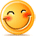:)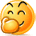:-)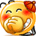:))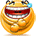=))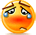:(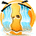:-(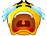:((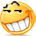:d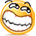:-d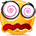@-)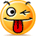:p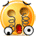:o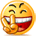:>)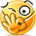(o)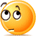[-(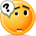:-?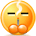(p)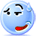:-s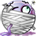(m)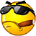8-)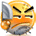:-t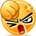:-b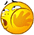b-(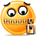:-#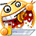=p~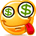:-\$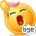(b)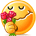(f)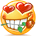x-)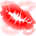(k)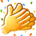(h)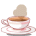(c)cheer